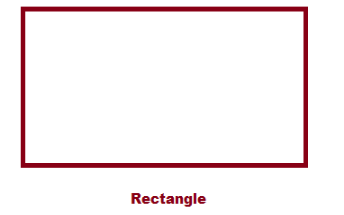# Rectangle Formula

Rectangle formulas include the formula for area, perimeter, and diagonal of a rectangle. To recall, a rectangle is a four sided polygon and the length of the opposite sides are equal. A rectangle is also called as an equiangular quadrilateral, as all the angles of a rectangle are right angled. A rectangle is a parallelogram with right angles in it. When the four sides of a rectangle are equal, then it is called a square.## Formula for Rectangle

There are mainly three formulas for rectangle – Perimeter, Area and Diagonal. The formulas for area, perimeter, and diagonal of a rectangle are:

Rectangle Formulas
Perimeter of a Rectangle Formula P = 2 (l + b)
Area of a Rectangle Formula A = l × b
Diagonal of a Rectangle Formula D =
$$\begin{array}{l}\sqrt{l^{2}+b^{2}}\end{array}$$

### Solved Questions Using Formulas of Rectangle

Question:

Find out the length of the rectangle if the area is 96 cm 2 and the breadth is 16 cm.

Solution:

As we know, the Area of a rectangle = l × b

Here the area is already given, so find the length of the rectangle.

So,96= l × 16

=>l= 96 ⁄ 16 i.e. Length = 6 cm